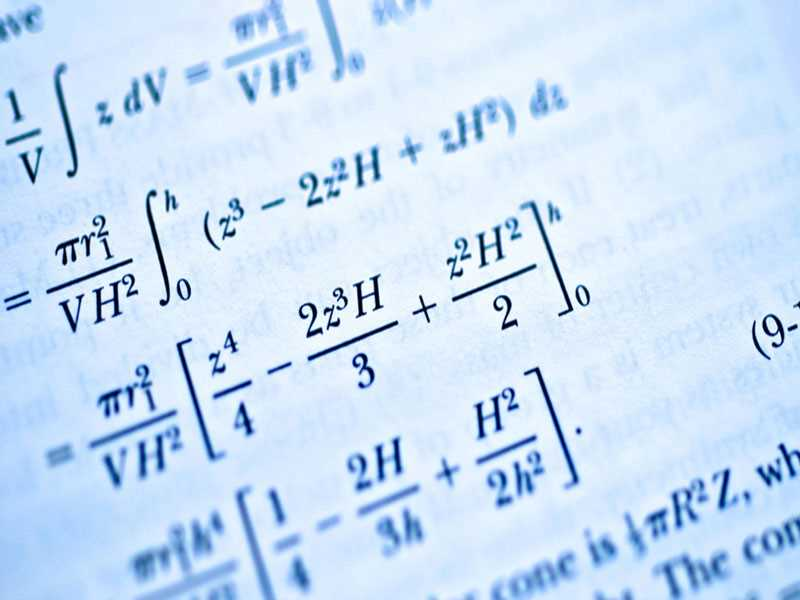# Mathematics

He has excellent academic and career opportunities that can satisfy your professional, personal and financial ambitions to the fullest extent. The analytical and organizational skills that the student learns during this study enable him to work in other sectors, including energy, health care, and even defense and military fields.

Mathematics is one of the most important and best disciplines in the world. It is a specialization of the Faculty of Science. It is taught in a large number of international universities. This specialization is very popular in the world and a large number of students are studying it.

Mathematics is defined as the science of abstract concepts and terms that indicate quantity and number, and this science indicates the amount of quantity and the amount of increase and decrease.

Mathematics has been defined by a number of scientists as a science of measurement, and mathematics is the language of science, and this specialization has played a major role in the development and progress of the world.

Mathematics is an ancient science known to ancient civilizations. Greek civilization has brought many mathematicians, many of whom came from Pythagoras, Thales, Euclid and Archimedes.

Mathematics has developed in the following years, and Arab civilization contributed to the development of this science greatly through the group of senior scientists and prominent Arab mathematicians Khwarizmi.

Mathematics study
Many students wish to study mathematics. In order to be able to study this discipline, students must obtain a high school diploma at a good rate.

The student must then study for a four-year bachelor's degree at the end of which he obtains a bachelor's degree in mathematics.

During the years of study the student receives a large number of sciences and courses that help him to understand this specialization and deepen it further.

If a student wants to complete a university degree in mathematics and master's degree in mathematics, he must achieve a good level at the bachelor's level. He must then study for a three-year master's degree in which he will study mathematics.

If a student wishes to study for a doctorate in mathematics, he must obtain a master's degree and must study for a doctorate for five years.

Characteristics and characteristics of mathematics
Mathematics is the queen of science because of its close association with other types of science. Mathematics is related to physics, chemistry, engineering of all kinds, medicine, the arts, and other sciences.

Mathematics is characterized by a large number of characteristics and features, including the abstract property, where the symbols and equations are used and these symbols are dealt with through non-physical relationships, and learning these symbols of the basics that every scientist of mathematics to master.

One of the features of mathematics is that a human being can not learn without a teacher, so the presence of the teacher is an essential element for learning and mastering mathematics.

An important feature of mathematics is the sequence, where understanding of mathematics requires a sequence of different steps. Solving a math problem requires a person to follow a number of steps in order to arrive at the ideal solution. If the student makes a mistake at some point, False.

Fields of work
Mathematics is one of the most important disciplines that provide students with a large number of opportunities to work. There are many fields where a mathematics graduate can work. Among the most prominent are teaching. A graduate of mathematics can work in schools and colleges. He can also work as a tutor. For mathematics, the student can work as a mathematics graduate as a tutor for this subject.

One of the areas where a mathematics graduate can work is technical jobs, and he can also work in financial services institutions.

A graduate of mathematics can work as a financial accountant in a large number of companies, and a graduate of this specialization can work in the field of research, physical and statistical institutions.

Objectives of Mathematics
Mathematics seeks to achieve many goals, among which are the following:

Understanding activities in society, helping to understand civilization, and following all developments in other sciences.
Mathematics contributes to solving the mysteries of a large number of natural phenomena and contributes to the recognition of the potential of the environment and society.
Mathematics contributes to the development of other areas of life such as science, arts, culture.
Mathematics contributes to increasing people's awareness of consumption, and thus a person can determine his daily, monthly, and yearly consumption.
Understanding the role of mathematics in life leads to progress in all fields of science and study.
Mathematics contributes to the creation of many values ​​in the person, such as truthfulness, system education, and accuracy.
Areas of Mathematics
Pure mathematics
Pure mathematics is one of the most important fields of mathematics, and it is concerned with the division of mathematics into a number of branches according to the main subject of the study.

Foundations of mathematics and philosophy
Mathematics can be divided into mathematical mathematics, engineering mathematics, analysis and algebra.

Applied Mathematics
Through this section are taught means and methods of sports used in various fields such as engineering, business, science and industry.

Turkey's distinguished universities in the field of mathematics
Istanbul Istanbul University
University of Sabanga
University of
Izmir University of Economics
University of Ted
University Bay